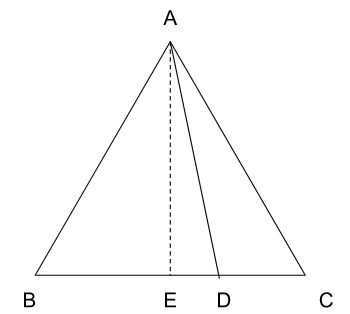# CAT 2022 Question Paper | Quant Slot 2

###### CAT Previous Year Paper | CAT Quant Questions | Question 21

CAT 2022 Quant was dominated by Arithmetic followed by Algebra. In Arithmetic, the questions were dominated by topics like Speed-time-distance, Mixture and Alligations. This year, there was a surprise. The questions from Geometry were relatively on the lower side as compared to the previous years. There were 8 TITA Qs this year. Overall this section was at a medium level of difficulty.

Question 21 : The length of each side of an equilateral triangle $$mathrm{ABC}$ is $3 $mathrm{~cm}$. Let $\mathrm{D}$ be a point on $\mathrm{BC}$ such that the area of triangle $\mathrm{ADC}$ is half the area of triangle $\mathrm{ABD}$. Then the length of $\mathrm{AD}$, in $\mathrm{cm}$, is 1. $\sqrt{6}$ 2. $\sqrt{5}$ 3. $\sqrt{8}$ 4. $\sqrt{7}$ ## Best CAT Online Coaching Try upto 40 hours for free Learn from the best! #### 2IIM : Best Online CAT Coaching. ### Video Explanation ## Best CAT Coaching in Chennai #### CAT Coaching in Chennai - CAT 2022Limited Seats Available - Register Now! ### Explanatory AnswerLet AE be the perpendicular dropped from A on to BC, AE = $\frac { \sqrt { 3 } } { 2 } 3$. E is the mid-point of BC. EC = 1.5 cm Ar$Δ ABD$ : Ar(Δ ADC) = 2 : 1
BD : DC = 2 : 1
DC = 1cm
ED = EC - DC = 1.5 cm - 1 cm = 0.5 cm
Δ AED is a right-angled triangle.